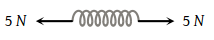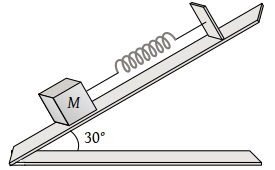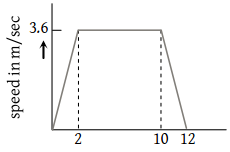A ball of mass 0.5 kg moving with a velocity of 2 m/sec strikes a wall normally and bounces back with the same speed. If the time of contact between the ball and the wall is one millisecond, the average force exerted by the wall on the ball is

(1) 2000 N

(2) 1000 N

(3) 5000 N

(4) 125 N

Concept Questions :-

Newton laws
High Yielding Test Series + Question Bank - NEET 2020

Difficulty Level:

A particle moves in the xy-plane under the action of a force F such that the components of its linear momentum p at any time t are ${p}_{x}=2\mathrm{cos}t$, ${p}_{y}=2\mathrm{sin}t$. The angle between F and p at time t is

(1) 90°

(2) 0°

(3) 180°

(4) 30°

Concept Questions :-

Newton laws
High Yielding Test Series + Question Bank - NEET 2020

Difficulty Level:

A satellite in force-free space sweeps stationary interplanetary dust at a rate $dM/dt=\alpha v$ where M is the mass, v is the velocity of the satellite and $\alpha$ is a constant. What is the deacceleration of the satellite

(1) $-2\alpha {v}^{2}/M$

(2) $-\alpha {v}^{2}/M$

(3) $+\alpha {v}^{2}/M$

(4) $-\alpha {v}^{2}$

Concept Questions :-

Variable mass system
High Yielding Test Series + Question Bank - NEET 2020

Difficulty Level:

Swimming is possible on account of

(1) First law of motion

(2) Second law of motion

(3) Third law of motion

(4) Newton's law of gravitation

Concept Questions :-

Newton laws
High Yielding Test Series + Question Bank - NEET 2020

Difficulty Level:

On a stationary sail-boat, air is blown at the sails from a fan attached to the boat. The boat will

(1) Remain stationary

(2) Spin around

(3) Move in a direction opposite to that in which air is blown

(4) Move in the direction in which the air is blown

Concept Questions :-

Newton laws
High Yielding Test Series + Question Bank - NEET 2020

Difficulty Level:

A man is standing at a spring platform. Reading of spring balance is 60 kg wt. If man jumps outside platform, then reading of spring balance

(1) First increases then decreases to zero

(2) Decreases

(3) Increases

(4) Remains same

Concept Questions :-

Newton laws
High Yielding Test Series + Question Bank - NEET 2020

Difficulty Level:

The tension in the spring is(1) Zero

(2) 2.5 N

(3) 5 N

(4) 10 N

Concept Questions :-

Spring force
High Yielding Test Series + Question Bank - NEET 2020

Difficulty Level:

A body of mass 5kg is suspended by a spring balance on an inclined plane as shown in figure. The spring balance measure(1) 50 N

(2) 25 N

(3) 500 N

(4) 10 N

Concept Questions :-

Spring force
High Yielding Test Series + Question Bank - NEET 2020

Difficulty Level:

A lift is going up. The total mass of the lift and the passenger is 1500 kg. The variation in the speed of the lift is as given in the graph. The tension in the rope pulling the lift at t = 11th sec will be(1) 17400 N

(2) 14700 N

(3) 12000 N

(4) Zero

High Yielding Test Series + Question Bank - NEET 2020

Difficulty Level:

A lift is going up. The total mass of the lift and the passenger is 1500 kg. The variation in the speed of the lift is as given in the graph. The height to which the lift takes the passenger is(1) 3.6 meters

(2) 8 meters

(3) 1.8 meters

(4) 36 meters